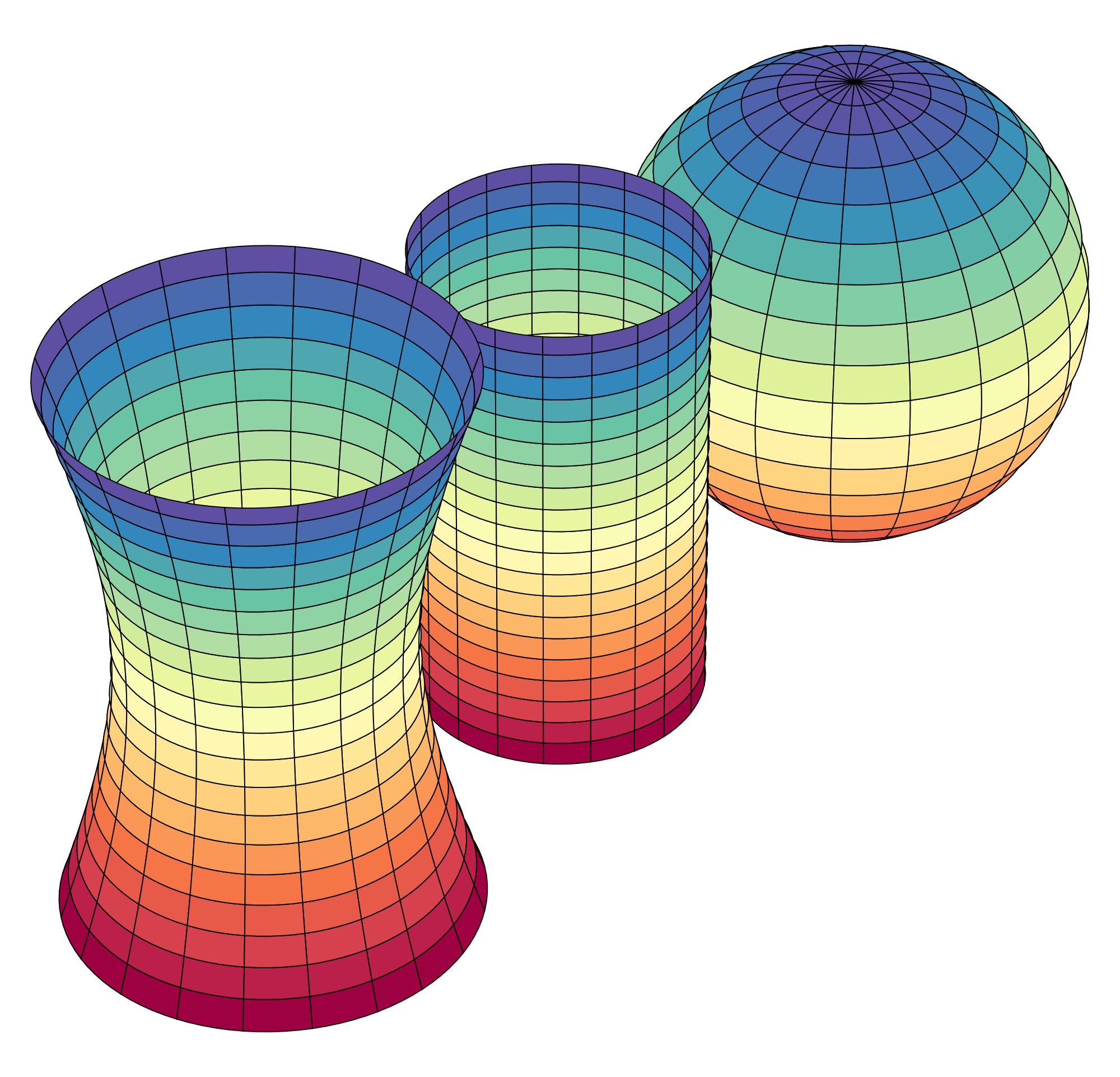# Calculus-Based Statistics with Calculus 2 Prerequisite

What are the course prerequisites for Calculus-Based Statistics = Probability Theory?

####Upper Division Math Courses

The only prerequisite for Calculus-Based Statistics is: Calculus II (Calculus 2) (and recommended Multivariable Calculus!)

As the name suggests, this is not the lower "baby statistics" course without Calculus. You need a very firm understanding of differential and integral Calculus to be able to engage Probability Theory.

Most of the topics in Probability Theory course are also found in the lower Statistics course, but the higher Probability Theory course uses derivatives and integrals to analyze the probability and statistics concepts and computations.

Multivariable Calculus is a good idea before Probability Theory, because some topics in Probability Theory use partial derivatives and multiple integrals - topics in Multivariable Calculus. While Multivariable Calculus is recommended, it is not required - learning these two differential and integral calculus constructions is not that difficult in the context of Probability Theory topics. So we keep Multivariable Calculus as a recommended course only.

So, for those students wishing to get ahead and get Probability Theory in their completed column in their academic plan, you do need to complete Calculus II first, which means also completing Calculus I first.

Here is a video about our Probability Theory course via Distance Calculus @ Roger Williams University:

## After AP Calculus for High School Students

Calculus-Based Statistics course can best be described as a "first course in the study of Statistics and Probablity Theory using Calculus".

This course has many names, all being equivalent:
• Probablity Theory
• Calculus-Based Statistics
• Second Course in Statistics for STEM

Our Probability Theory course differs from a classroom/textbook-based course in that we employ Mastery Learning so that you complete all assignments at 100% to assure competancy, as well as our curriculum shifting the course to a laboratory-style course, where theorem/lemma/proof type exposition is replaced by running experiments in Mathematica as you would in a science laboratory to empirically deduce the concepts and behaviors of Probability Theory, both solvable (classically) via hand-based techiques, as well as studying Probability Theory that can only be solved and investigated graphically and numerically using a computer. The Probability Theory curriculum is highly visual and based upon observations of experiments run in Mathematica or LiveMath.

At Distance Calculus, we call our "Calculus-Based Statistics" course as Probability Theory - DMAT 311 - 3 credits.

Below are some links for further information about the Calculus-Based Statistics course via Distance Calculus @ Roger Williams University.

## Distance Calculus - Student ReviewsDate Posted: Apr 29, 2020
Review by: Harlan E.
Courses Completed: Calculus I, Calculus II
Review: I did not do well in AP Calculus during my senior year in high school. Instead of trying to cram for the AP exam, I decided to jump ship and go to Distance Calculus to complete Calculus I. This was awesome! I finished Calculus I in about 6 weeks, and then I kept going into Calculus II. I started as a freshman at UCLA with both Calculus I and II done!
Transferred Credits to: University of California, Los AngelesDate Posted: May 3, 2020
Review by: Andris H.
Courses Completed: Applied Calculus
Review: I found out from my MBA program that I needed to finish calculus before starting the MBA. They told me 3 weeks before term started! I was able to finish Applied Calculus from Distance Calculus. Definitely a great class. Thanks Distance Calculus!
Transferred Credits to: SUNY Stony BrookDate Posted: Apr 10, 2020
Review by: Benjamin T.
Courses Completed: Calculus I
Review: This course provided an excellent chance to learn about Calculus...again. I took calculus in high school, but I learned so much more with this course! It does take a good amount of time to do all the lessons, so definitely keep on top of them, but all the exercises helped me to really understand the material. And the nice thing is you can do it on your own time at home.
Transferred Credits to: Western University of Health Sciences: College of Optometry